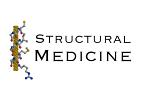# Protein Crystallography CourseCourse Homepage   Basic: 1 2 3 4 5 6   Advanced: 7 8 9 10 11 12 13 14 15 16 17 18 19 20 21 22

# The phase problem

We've seen that, when waves are diffracted from a crystal, they give rise to diffraction spots. Each diffraction spot corresponds to a point in the reciprocal lattice and represents a wave with an amplitude and a relative phase. But really what happens is that photons are reflected from the crystal in different directions with a probability proportional to the square of the amplitude of this wave. We count the photons, and we lose any information about the relative phases of different diffraction.

The figure below shows again how the phase and amplitude of the overall scattered wave arise from the individual scattered waves. Two Bragg planes are shown, together with four atoms. The relative phase (from 0 to 360 degrees) depends on the relative distance of the atoms between the planes that define a phase angle of zero. The atoms and their contributions to the scattering (represented as vectors) are shown in matching colours. The overall scattered wave is represented by a black vector, which is the sum of the other vectors.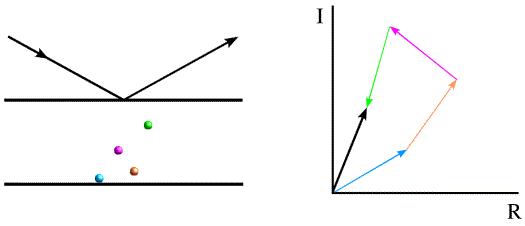The vector (amplitude and phase or, more properly, the complex number) representing the overall scattering from a particular set of Bragg planes is termed the structure factor, and it is usually denoted F. (The use of bold font indicates that it is a vector or complex number.)

It turns out (for reasons beyond the present discussion) that the structure factors for the various points on the reciprocal lattice correspond to the Fourier transform of the electron density distribution within the unit cell of the crystal. A very convenient property of the Fourier transform is that it is reversible; if you apply an inverse Fourier transform to the structure factors, you get back the electron density. You might want to look at Kevin Cowtan's Interactive Structure Factor Tutorial again to remind yourself how this works.

So we measure a diffraction pattern, take the square roots of the intensities, and we're stuck: if we knew the phases we could simply compute a picture of the molecule, but we've lost the information in the experiment! This is the phase problem, and a large part of crystallography is devoted to solving it.

# How to solve the phase problem

## Guess the structure

In the beginning, crystallographers worked on the structures of simple molecules and they could often make a good guess of the conformation of a molecule and even how it might pack in the crystal lattice. The guesses could be tested by calculating a diffraction pattern and comparing it to the observed one. If a guess places the atoms in about the right place, then the calculated phases will be approximately correct and a useful electron density map can be computed by combining the observed amplitudes with the calculated phases. If the model is reasonably accurate, such a map will show features missing from the model so that the model can be improved. You can remind yourself how this works by looking at Kevin Cowtan's cats.

For proteins, we can only guess what the structure will look like if we've already seen a closely-related protein structure before. And then we still have to work out how it is oriented and where it is located in the unit cell. The technique to use prior structural information, called molecular replacement, is discussed below after the Patterson function, which provides a way to understand it.

## Patterson function

Remember that, if we carry out an inverse Fourier transform of the structure factors (amplitudes and phases), we get a picture of the electron density. Patterson asked the question of what we would get if we took a Fourier transform of the intensities (amplitudes squared) instead, which would only require the measured data. It turns out that the resulting map, which is now called a Patterson function or Patterson map, has some very interesting and useful features.

We won't go into the math here, but it turns out that the Patterson function gives us a map of the vectors between atoms. In other words, if there is a peak of electron density for atom 1 at position x1 and a peak of electron density for atom 2 at position x2, then the Patterson map will have peaks at positions given by x2-x1 and x1-x2. The height of the peak in the Patterson map is proportional to the product of the heights of the two peaks in the electron density map. The figure below illustrates a Patterson map corresponding to a cell with one molecule. It demonstrates that you can think of a Patterson as being a sum of images of the molecule, with each atom placed in turn on the origin. Because for each vector there is one in the opposite direction, the same Patterson map is also a sum of inverted images of the molecule, as shown in the bottom representation.For relatively small numbers of atoms, it is possible to work out the original positions of the atoms that would give rise to the observed Patterson peaks. This is called deconvoluting the Patterson. But it quickly becomes impossible to deconvolute a Patterson for larger molecules. If we have N atoms in a unit cell and the resolution of the data is high enough, there will be N separate electron density peaks in an electron density map. In a Patterson map, each of these N atoms has a vector to all N atoms, so that there are N2 vectors. N of these will be self-vectors from an atom to itself, which will accumulate as a big origin peak, but that still leaves N2-N non-origin peaks to sort out. If N is a small number, say 10, then we will have a larger but feasible number of non-origin Patterson peaks to deal with (90 for N=10). But if N were 1000, which would be more in the range seen for protein crystals, then there would be 999,000 non-origin Patterson peaks. And even at high resolution the protein atoms are barely resolved, so there's no chance that the Patterson peaks will be resolved from each other!

Nonetheless, the Patterson function becomes useful as part of other methods to solve structures, as we will soon see.

## Molecular replacement

Molecular replacement can be used when you have a good model for a reasonably large fraction of the structure in the crystal. The level of resemblance of two protein structures correlates well with the level of sequence identity, which means that you can get a good idea of whether or not molecular replacement will succeed before even trying it. As a rule of thumb, molecular replacement will probably be fairly straightforward if the model is fairly complete and shares at least 40% sequence identity with the unknown structure. It becomes progressively more difficult as the model becomes less complete or shares less sequence identity.

To carry out molecular replacement, you need to place the model structure in the correct orientation and position in the unknown unit cell. To orient a molecule you need to specify three rotation angles and to place it in the unit cell you need to specify three translational parameters. So if there is one molecule in the asymmetric unit of the crystal, the molecular replacement problem is a 6-dimensional problem. It turns out that it is usually possible to separate this into two 3D problems. A rotation function can be computed to find the three rotation angles, and then the oriented model can be placed in the cell with a 3D translation function.

An understanding of the rotation and translation functions can be obtained most easily by considering the Patterson function. Even though the vectors are unresolved for a structure the size of a protein, the way that they accumulate can provide a signature for a protein structure. The vectors in the Patterson map can be divided into two categories. Intramolecular vectors (from one atom in the molecule to another atom in the same molecule) depend only on the orientation of the molecule, and not on its position in the cell, so these can be exploited in the rotation function. Intermolecular vectors depend both on the orientation of the molecule and on its position so, once the orientation is known, these can be exploited in the translation function.

Crystal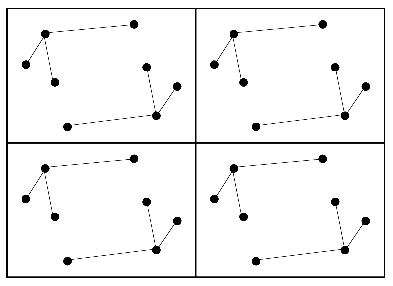Patterson map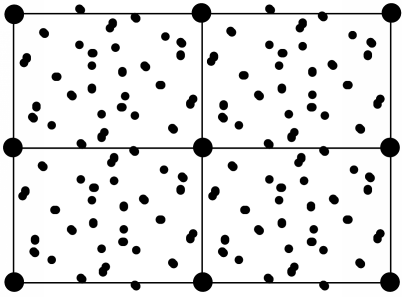Intramolecular vectors before rotation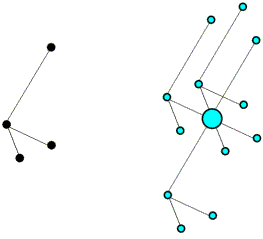Colour-coded Patterson map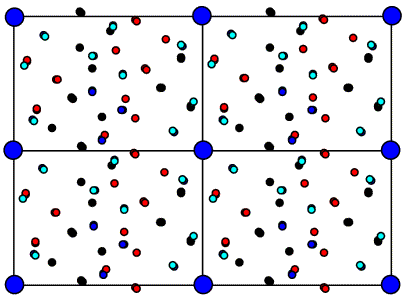Intramolecular vectors after rotation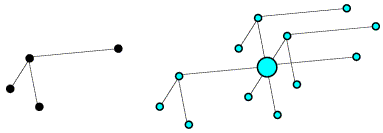On average, the intramolecular vectors will be shorter than the intermolecular vectors, so the rotation function can be computed using only the part of the Patterson map near the origin.

## Direct methods

We won't go into detail here, but it turns out that if you assume that a crystal is made up of similarly-shaped atoms that all have positive electron density, then there are statistical relationships between sets of structure factors. These statistical relationships can be used to deduce possible values for the phases. Direct methods exploit such relationships, and can be used to solve small molecule structures. Unfortunately, the statistical relationships become weaker as the number of atoms increases, and direct methods are limited to structures with, at most, a few hundred atoms in the unit cell. Although there are developments that push these limits, particularly for crystals that diffract to very high resolution (1.2Å or better), direct methods are not generally applicable to the vast majority of crystal structures. However, they do become useful in the context of experimental phasing methods, such as isomorphous replacement and anomalous dispersion, as discussed below.

## Multiple isomorphous replacement

In isomorphous replacement, the idea is to make a change to the crystal that will perturb the structure factors and, by the way that they are perturbed, to make some deductions about possible phase values. It is necessary to be able to explain the change to the crystal with only a few parameters, which means that we have to use heavy atoms (heavy in the sense that they have a large atomic number, i.e. many electrons). The figure below illustrates the effect of adding a heavy atom to the structure considered above.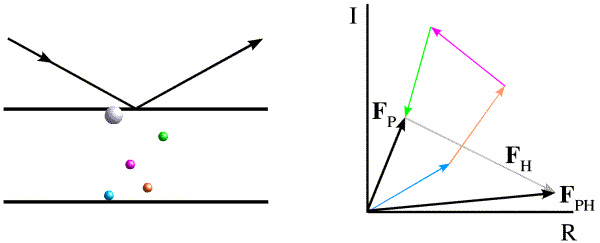The introduction of a heavy atom will change the scattered intensity significantly. One reason for this is that "heavy" atoms contribute disproportionately to the overall intensity. As you can see from the figure, the contributions from the lighter atoms will tend to cancel out, because they will scatter with different phase angles. On the other hand, all of the electrons in a heavy atom will scatter essentially in phase with one another. Because of this effect, different atoms contribute to the scattered intensity in proportion to the square of the number of electrons they contain. For example, a uranium atom contains 15 times as many electrons as a carbon atom, so its contribution to the intensity will be equivalent to that of 225 carbon atoms. As a result, the change in intensity from the addition of 1 uranium atom to a protein of 20kDa is easily measured.

If we have two crystals, one containing just the protein (native crystal) and one containing in addition bound heavy atoms (derivative crystal), we can measure diffraction data from both. The differences in scattered intensities will largely reflect the scattering contribution of the heavy atoms, and these differences can be used (for instance) to compute a Patterson map. Because there are only a few heavy atoms, such a Patterson map will be relatively simple and easy to deconvolute. (Alternatively, direct methods can be applied to the intensity differences.) Once we know where the heavy atoms are located in the crystal, we can compute their contribution to the structure factors.

This allows us to make some deductions about possible values for the protein phase angles, as follows. First, note that we have been assuming that the scattering from the protein atoms is unchanged by the addition of heavy atoms. This is what the term "isomorphous" (= "same shape") refers to. ("Replacement" comes from the idea that heavy atoms might be replacing light salt ions or solvent molecules.) If the heavy atom doesn't change the rest of the structure, then the structure factor for the derivative crystal (FPH) is equal to the sum of the protein structure factor (FP) and the heavy atom structure factor (FH), or

FPH = FP + FH

If we remember that the structure factors can be thought of as vectors, then this equation defines a triangle. We know the length and orientation of one side (FH), and the lengths of the other two sides. As shown in the figure below, there are two ways to construct such a triangle, which means that there are two possible phases for FP.There is another way, called the Harker construction, to show the two possible phases. This ends up being more useful because it generalises nicely when there is more than one derivative. First we draw a circle with a radius equal to the amplitude of FP (denoted |FP|), centered at the origin (blue in the figure below). The circle indicates all the vectors that would be obtained with all the possible phase angles for FP. Next we draw a circle with radius |FPH| centered at a point defined by -|FH| (magenta in the figure below). All of the points on the magenta circle are possible values for FP (magnitude and phase) that satisfy the equation FPH = FH + FP while agreeing with the measured amplitude |FPH|. There are two possible values for FP that agree with the both measured amplitudes and with the heavy atom model.In principle, the twofold phase ambiguity can be removed by preparing a second derivative crystal with heavy atoms that bind at other sites. The information from the second derivative is illustrated in green below, showing that only one phase choice is consistent with all the observations. The need for multiple derivatives to obtain less ambiguous phase information is the reason for the term "multiple" in "multiple isomorphous replacement".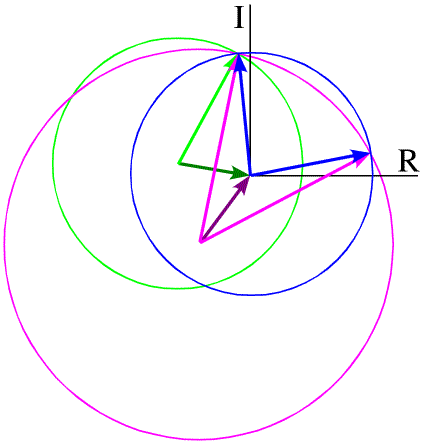These figures have all been drawn assuming that there are no errors in the model for the heavy atoms in the derivative crystal, no error in measuring the amplitudes of the structure factors, and also assuming that the two crystals are perfectly isomorphous. The effect of these sources of uncertainty is to smear out the circles, so that the regions of overlap are much more diffuse and much more ambiguity remains.

## Anomalous dispersion

Most electrons in the atoms that make up a crystal will interact identically with X-rays. If placed at the origin of the crystal, they will diffract with a relative phase of zero. Because of this, pairs of diffraction spots obey Friedel's law, which is illustrated below. On the left, the black arrows indicate a diffraction event from the top of the planes. The atoms contribute to the diffraction pattern with phases determined by their relative distances from the planes, as indicated by the colour-coded arrows on the right. The red arrows on the left indicate a very similar diffraction event, but from the bottom of the same planes. The angles of incidence and reflection are the same, and all that is different is which side of the planes we're looking at. If the black arrows define planes with Miller indices (h k l), the same planes are defined from the other side with Miller indices (-h -k -l). The reflection with indices (-h -k -l) is referred to as the Friedel mate of (h k l). Atoms will contribute with the same phase shift, but where the phase shifts were positive they will now be negative. This is illustrated on the right with the red arrows on the bottom, each of which has the opposite phase of the coloured arrows on the top. The effect of reversing the phases is to reflect the picture across the horizontal axis.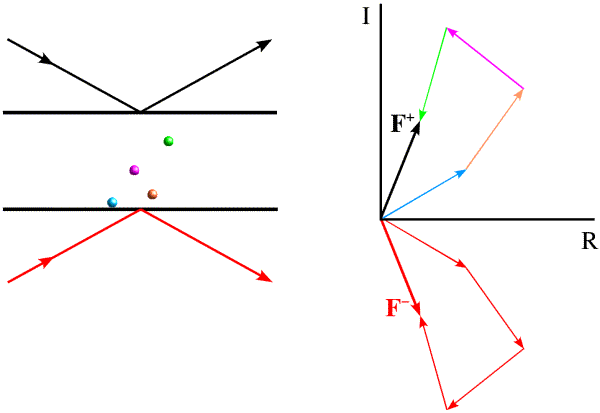Remember the picture we had of the electric field of the electromagnetic wave inducing an oscillation in the electrons. You may have studied the behaviour of driven oscillators in physics. As long as the frequency of oscillation is very different from the natural frequency of oscillation, the electrons will all oscillate with the same phase. This is true of most electrons in a crystal. But if it is similar to the natural frequency of oscillation, then there will be a small shift in both the amplitude and phase of the induced oscillation. This is true for some inner shell electrons in some atoms, where the X-ray photon energy is close to a transition energy. (Such transitions are used, in fact, to generate X-rays with a characteristic wavelength. We often use a particular transition of electrons in copper.) The shift in amplitude and phase is called anomalous scattering.

The phase shift in anomalous scattering leads to a breakdown of Friedel's law, as illustrated in the figure below. Now we have added a heavy atom with an anomalous scattering component. It is convenient to represent the phase shift by adding a vector at 90 degrees to the normal scattering for the heavy atom. Significantly, this vector is at +90 degrees from the contribution from the anomalous scattering, regardless of which of the two Friedel mates we are looking at. And this causes the symmetry to break down.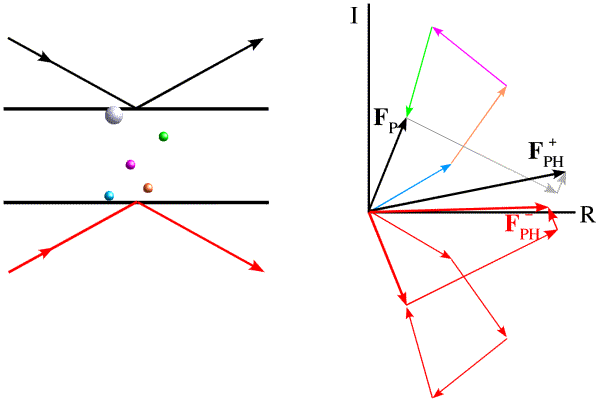The effect is easier to see (and to use) if we take the Friedel mate and reverse the sign of its phase, i.e. reflect it across the horizontal axis. (Thinking of the structure factor as a complex number, this means that we reverse the sign of the imaginary component, the result of which is called the complex conjugate, indicated with an asterisk.)Now we can see that the effect of anomalous scattering has been to make the amplitudes of the Friedel mates different. You can see that, if we have a model for the anomalous scatterers in the crystal, we can draw vectors for their contribution to the structure factors for the Friedel mates and construct a Harker diagram, as in the case of MIR.

The anomalous scattering effect depends on the frequency of oscillation being similar to the natural frequency for the atom. So clearly the strength of the anomalous scattering effect depends on the wavelength of the X-rays, which will change both the normal scattering and the out-of-phase scattering of the anomalous scatterers. By collecting data at several wavelengths near the absorption edge of an element in the crystal, we can obtain phase information analogous to that obtained from MIR. This technique is called MAD, for multiple-wavelength anomalous dispersion. One popular way to use MAD is to introduce selenomethionine in place of methionine residues in a protein. The selenium atoms (which replace the sulfur atoms) have a strong anomalous signal at wavelengths that can be obtained from synchrotron X-ray sources.

# How to improve phases

Depending on the quality of the phasing experiment (quality of diffraction data, quality of protein model for molecular replacement or heavy atom model for isomorphous replacement or anomalous dispersion), there can be rather large errors in the phases and thus in the electron density maps. Over the last twenty years or so, a variety of techniques have been developed to improve the phases. These methods are mostly based on the idea that we know something about the characteristics of a good electron density map, and if we change the map to look more like a good one, phases computed from the this map will be more accurate than the original phases.

## Density modification

The term "density modification" is used to describe a number of techniques in which the density map is modified to have the features we would expect from a good map.

Solvent flattening. It turns out that, in a typical protein crystal, about half of the volume is occupied by well-ordered protein molecules while the other half is occupied by disordered solvent. We know that the disordered solvent should have flat, featureless electron density, so if there are features in the solvent region they are probably the result of phase errors. Intuitively, if the density map is modified so that the solvent region is flattened, the corresponding phases will be more accurate. (As we will see later, another way of looking at this is that solvent flattening uses phase information from other structure factors to improve the phase information of a particular structure factor.) To carry out solvent flattening, the phases have to be at least good enough to see the boundaries between the disordered solvent and the ordered protein. Fortunately, there are algorithms to define the boundaries automatically; the first of these was proposed by B.C. Wang.

Averaging. Frequently proteins crystallise with more than one copy in the unique part (asymmetric unit) of the unit cell of the crystal. In other cases, proteins crystallise in different crystal forms. For the most part, the structure of a protein is fairly fixed and does not depend much on its environment. So we expect that when the same protein appears in different places in an electron density map (or in maps from different crystals), the density should be more or less the same in each copy. As for solvent flattening, if they differ it is probably because of errors in the phases. By averaging the density, we cancel out some of the random errors and thereby increase the accuracy of the corresponding phases.

Histogram matching. This one is slightly less obvious. Proteins are made up of the same atom types with the same sorts of relative distances and, as a result, the same kinds of density values are seen in electron density maps for different proteins. If a map in the protein region does not have the distribution of low and high densities that one expects, this is probably because of phase errors. By altering the distribution of density values to match what we expect (with an algorithm called histogram matching), the corresponding phases are again made more accurate.

## Automated fitting and refinement

This can be thought of as another form of density modification. We know that protein structures are made up of atoms. If the density can be interpreted in terms of an atomic model (and the atoms are put more or less in the right place), the density distribution will be closer to the truth and the corresponding phases will yet again be more accurate. Because we know a lot about how the atoms are arranged relative to each other (bond lengths, bond angles, the chemical connectivity defined by the amino acid sequence), we can exploit a lot of information in building an atomic model. If it is possible to make a good start, then the approach can be applied iteratively: model building into a density map is followed by refinement to gain better agreement with the observed diffraction data, then the new improved phases can be used to compute a new, better density map. Optionally, other density modification techniques (such as averaging and solvent flattening) can be applied before a new cycle is started with the building of a new model.